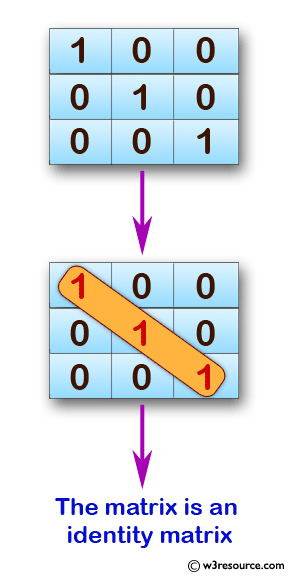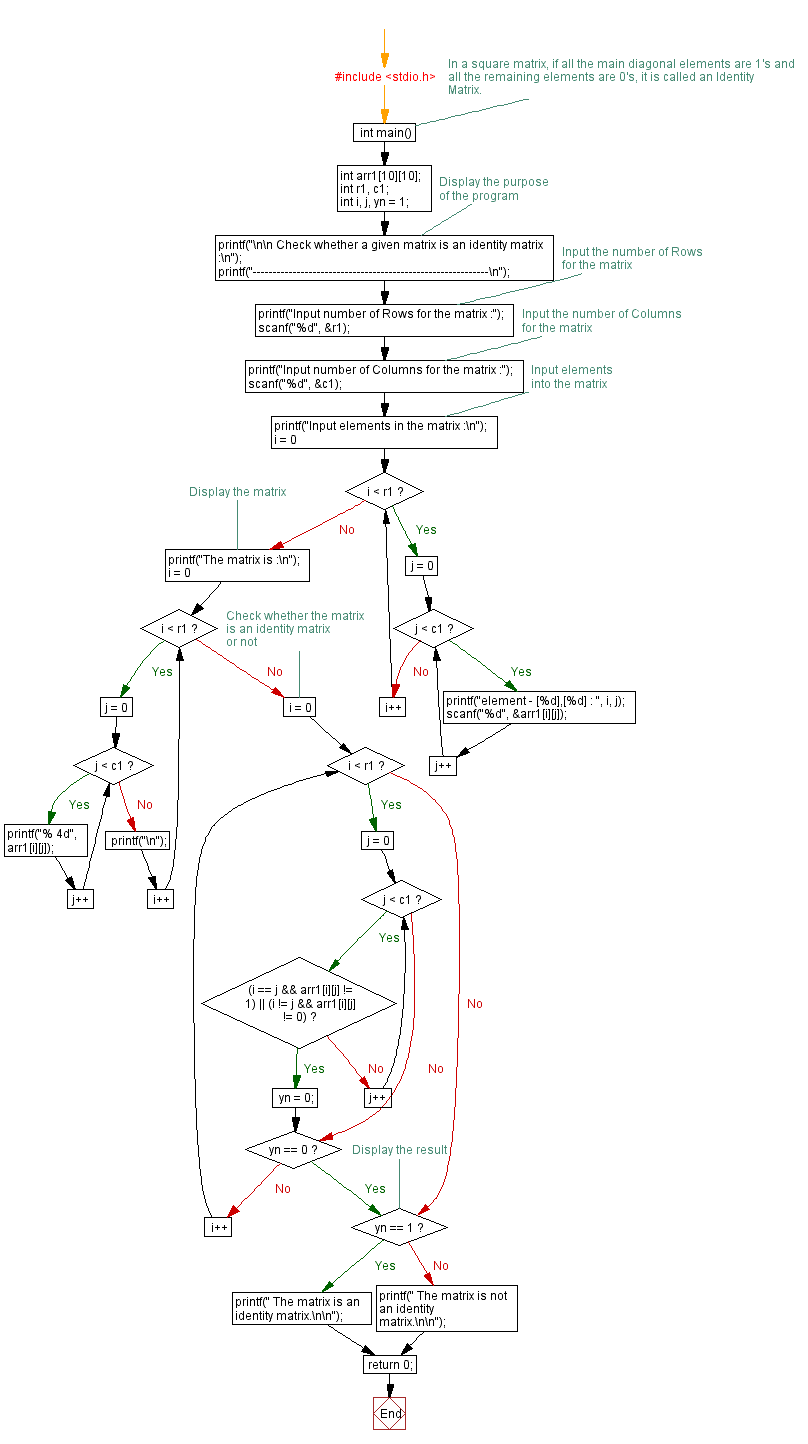﻿ C exercises: Check whether a given matrix is an identity matrix - w3resource# C Exercises: Check whether a given matrix is an identity matrix

## C Array: Exercise-31 with Solution

Write a program in C to check whether a given matrix is an identity matrix.

Pictorial Presentation:Sample Solution:

C Code:

``````#include <stdio.h>
//In a square matrix if all the main diagonal elements are 1's and
//all the remaining elements are 0's is called an Identity Matrix.

void main()
{
int arr1;
int r1,c1;
int i, j, yn =1;

printf("\n\n Check whether a given matrix is an identity matrix :\n ");
printf("-----------------------------------------------------------\n");

printf("Input number of Rows for the matrix :");
scanf("%d", &r1);
printf("Input number of Columns for the matrix :");
scanf("%d",&c1);
printf("Input elements in the first matrix :\n");
for(i=0;i<r1;i++)
{
for(j=0;j<c1;j++)
{
printf("element - [%d],[%d] : ",i,j);
scanf("%d",&arr1[i][j]);
}
}
printf("The matrix is :\n");
for(i=0;i<r1;i++)
{
for(j=0;j<c1 ;j++)
printf("% 4d",arr1[i][j]);
printf("\n");
}

for(i=0; i<r1; i++)
{
for(j=0; j<c1; j++)
{
if(arr1[i][j] != 1 && arr1[j][i] !=0)
{
yn = 0;
break;
}
}
}

if(yn == 1 )
printf(" The matrix is an identity matrix.\n\n");
else
printf(" The matrix is not an identity matrix.\n\n");
}
```
```

Sample Output:

``` Check whether a given matrix is an identity matrix :
-----------------------------------------------------------
Input number of Rows for the matrix :3
Input number of Columns for the matrix :3
Input elements in the first matrix :
element - , : 1
element - , : 0
element - , : 0
element - , : 0
element - , : 1
element - , : 0
element - , : 0
element - , : 0
element - , : 1
The matrix is :
1   0   0
0   1   0
0   0   1
The matrix is an identity matrix.
```

Flowchart :C Programming Code Editor:

Improve this sample solution and post your code through Disqus.

What is the difficulty level of this exercise?

﻿